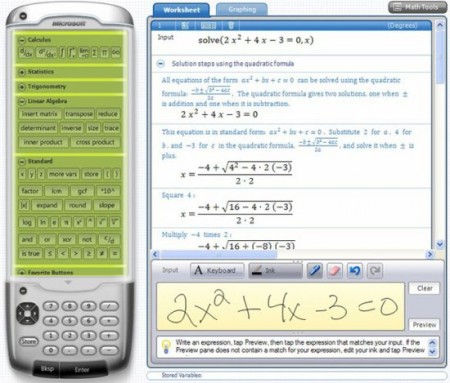Editor Ratings:
User Ratings:
[Total: 0 Average: 0]

Microsoft Mathematics is a free math problem solver that helps students by giving a complete step-by-step solution to algebraic equations. In addition, Microsoft Mathematics also brings a graphic calculator to you that plots in 2D & 3D and various other useful options.

This free math problem solver basically provides students a set of mathematical tools which helps them to complete their school work easily and quickly.With Microsoft Mathematics, students can be taught to solve difficult to difficult equations in a simple step-by-step manner. This helps them get better learning of basic fundamental concepts in physics, chemistry, pre-algebra, algebra, calculus, and trigonometry. The design is just like a hand-held calculator.

## Some key features of this Math Problem Solver:

• Students can learn step wise as to how to solve difficult mathematical problems.
• New improved three-dimensional color graphs can better illustrate problems and concepts.
• Full fledged library consists of equations and formulas
• More than 100 basic equations and formulae to help identify and apply equations.
• Unit conversion tool

Check out our post on Geogebra Maths Learning Software.

• Go onto the website.
• In front of the second one which is 17.0MB, Click on “Download”
• Click on Save